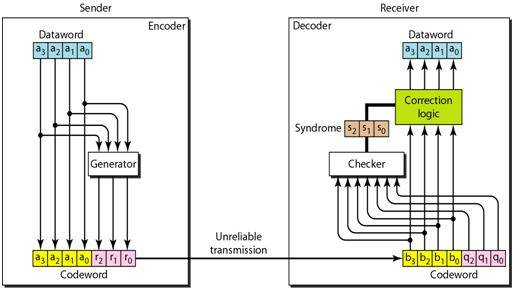# Implementation of Hamming code

By | July 10, 2016
• This post gives an idea on how to implement Hamming Code. Hamming code pattern is mainly used for detection of errors in bits.

## Objectives:

• The main objective is, detection of single bit error, burst error and to correct by using the suitable methodology (by hamming distance concept).
• The purpose of this project is to overcome unreliable transmission of data during the transmission from the encoder to the decoder.
• It involves block coding, finding the hamming distance between to existing code words, redundancy, error correction, parity check code.

## Description:

The theory of error detecting and correcting codes is that branch of engineering and mathematics which deals with the reliable transmission and storage of data. Information media are not 100% reliable in practice, in the sense that noise (any form of interference) frequently causes data to be distorted. To deal with this undesirable but inevitable situation, self-correcting codes are constructed by combining data bits with a number of redundant check-bits, which together are referred to as code words.  By adding a single bit (as in Parity Checking) along with a given number of data-bits, half of the possible code words become valid and half invalid.  This is referred to as a code that has Hamming Distance 2 (two single bit flips are required to get from one calid code to another). This particular approach, using Hamming Distance, is the minimum requirement for self-error correction and is commonly referred to as a Hamming Code.

## Preliminaries:

a. Hamming distance H(a,b) = #number of bit positions in which a differs from b, i.e., the number of 1’s in an XOR. Hamming distance of a code is the minimum over all pairs of distinct code words of the Hamming distance between them, i.e.,

H(code) = min {H(a,b) | a<>b and a, b in code}

b. Error correction – enough redundancy is transmitted in the code that errors can be corrected by the receiver without retransmission.

c. Error detection is the detection of errors caused by noise or other impairments during transmission from the transmitter to the receiver.

d. d-bit error detection requires a code with Hamming distance (d+1).
d-bit error correction requires a code with Hamming distance (2d+1).

## Tools  Required:

• Encoder.
• Decoder.
• cyclic redundancy check (CRC) or polynomial code checksum.
• linear block codes.
• Two single bit Flip-Flop.

## Future Scope:

In the field of artificial intelligence, where there is a need to search through large state spaces, there is the notion of using evaluation functions to heuristically search a large space in a hill climbing or the best-first search fashion. Since Hamming distance is an easy-to-define metric, it is used to search the state space for design flaws.

Hamming code is an easy and efficient technique, which can only detect and correct a single bit error. However. it can also be used to detect a burst error (A burst error means that 2 or more bits in the data unit have changed), but by applying the different technique.

The structure of the encoder and decoder for a Hamming codeStructure of the encoder and decoder for a Hamming code

#### Following is the code in C language to implement hamming code:

Program to implement 7-bit hamming code:Author: Ravi Bandakkanavar

A Techie, Blogger, Web Designer, Programmer by passion who aspires to learn new Technologies every day. It has been 8 years since I have been publishing articles and enjoying every bit of it. I want to share the knowledge and build a great community with people like you.

Suggested articles for you:

## 4 thoughts on “Implementation of Hamming code”

1.sahilvaghasiya9687gmailcom

using this formula 2^r>=m+r+1 then create program

2.KD7

This is correct code for 7bit hamming code please refer this:——–
#include

void main() {
int data;
int dataatrec,c,c1,c2,c3,i;

printf(“Enter 4 bits of data one by one\n”);
scanf(“%d”,&data);
scanf(“%d”,&data);
scanf(“%d”,&data);
scanf(“%d”,&data);

data=data^data^data;
data=data^data^data;
data=data^data^data;

printf(“\nEncoded data is\n”);
for(i=0;i<7;i++)
printf("%d",data[i]);

printf("\n\nEnter received data bits one by one\n");
for(i=0;i<7;i++)
scanf("%d",&dataatrec[i]);

c1=dataatrec^dataatrec^dataatrec^dataatrec;
c2=dataatrec^dataatrec^dataatrec^dataatrec;
c3=dataatrec^dataatrec^dataatrec^dataatrec;
c=c3*4+c2*2+c1 ;

if(c==0 && dataatrec==data && dataatrec==data && dataatrec==data ) {
printf("\nNo error while transmission of data\n");
}
else {
printf("\nError on position %d",c);

printf("\nData sent : ");
for(i=0;i<7;i++)
printf("%d",data[i]);

for(i=0;i<7;i++)
printf("%d",dataatrec[i]);

printf("\nCorrect message is\n");

if(dataatrec[7-c]==0)
dataatrec[7-c]=1;
else
dataatrec[7-c]=0;

for (i=0;i<7;i++) {
printf("%d",data[i]);
}
}
}

1.Ravi Bandakkanavar Post author

Thanks for the code Kedar. Is it tested one?

3.Dhrisya krishna

How this error can be implemented as application level in project